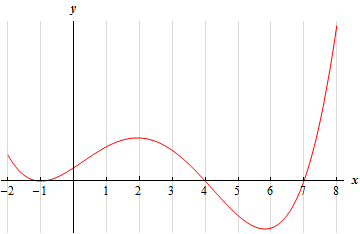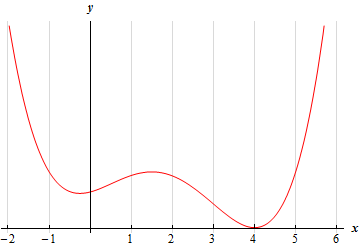Paul's Online Notes
Home / Calculus I / Applications of Derivatives / The Shape of a Graph, Part II
Show Mobile Notice Show All Notes Hide All Notes
Mobile Notice
You appear to be on a device with a "narrow" screen width (i.e. you are probably on a mobile phone). Due to the nature of the mathematics on this site it is best views in landscape mode. If your device is not in landscape mode many of the equations will run off the side of your device (should be able to scroll to see them) and some of the menu items will be cut off due to the narrow screen width.
Assignment Problems Notice
Please do not email me to get solutions and/or answers to these problems. I will not give them out under any circumstances nor will I respond to any requests to do so. The intent of these problems is for instructors to use them for assignments and having solutions/answers easily available defeats that purpose.

If you are looking for some problems with solutions you can find some by clicking on the "Practice Problems" link above.

### Section 4.6 : The Shape of a Graph, Part II

For problems 1 & 2 the graph of a function is given. Determine the intervals on which the function is concave up and concave down.

1.2.For problems 3 – 5 the graph of the 2nd derivative of a function is given. From this graph determine the intervals in which the function is concave up and concave down.

1.2.3.For problems 6 – 18 answer each of the following.

1. Determine the intervals on which the function is concave up and concave down.
2. Determine the inflection points of the function.

1. $$f\left( x \right) = {x^3} + 9{x^2} + 24x - 6$$
2. $$Q\left( t \right) = {t^4} - 2{t^3} - 120{t^2} - 84t + 35$$
3. $$h\left( z \right) = 3{z^5} - 20{z^4} + 40{z^3}$$
4. $$g\left( w \right) = 5{w^4} - 2{w^3} - 18{w^2} + 108w - 12$$
5. $$g\left( x \right) = 10 + 360x + 20{x^4} + 3{x^5} - {x^6}$$
6. $$A\left( x \right) = 9x - 3{x^2} - 160\sin \left( {\frac{x}{4}}} \right$$ on $$\left[ { - 20,11} \right]$$
7. $$f\left( x \right) = 3\cos \left( {2x} \right) - {x^2} - 14$$on $$\left[ {0,6} \right]$$
8. $$h\left( t \right) = 1 + 2{t^2} - \sin \left( {2t} \right)$$ on $$\left[ { - 2,4} \right]$$
9. $$R\left( v \right) = v{\left( {v - 8} \right)^{{\frac{1}{3}}}}$$
10. $$g\left( x \right) = \left( {x - 1} \right){\left( {x + 3} \right)^{{\frac{2}{5}}}}$$
11. $$f\left( x \right) = {{\bf{e}}^{4\,x}} - {{\bf{e}}^{ - \,x}}$$
12. $$h\left( w \right) = {w^2}{{\bf{e}}^{ - w}}$$
13. $$A\left( w \right) = {w^2} - \ln \left( {{w^2} + 1} \right)$$

For problems 19 – 33 answer each of the following.

1. Identify the critical points of the function.
2. Determine the intervals on which the function increases and decreases.
3. Classify the critical points as relative maximums, relative minimums or neither.
4. Determine the intervals on which the function is concave up and concave down.
5. Determine the inflection points of the function.
6. Use the information from steps (a)(e) to sketch the graph of the function.

1. $$f\left( x \right) = 10 - 30{x^2} + 2{x^3}$$
2. $$G\left( t \right) = 14 + 4{t^3} - {t^4}$$
3. $$h\left( w \right) = {w^4} + 4{w^3} - 18{w^2} - 9$$
4. $$g\left( z \right) = 10{z^3} + 10{z^4} + 3{z^5}$$
5. $$f\left( z \right) = {z^6} - 9{z^5} + 20{z^4} + 10$$
6. $$Q\left( t \right) = 3t - 5\sin \left( {2t} \right)$$ on $$\left[ { - 1,4} \right]$$
7. $$g\left( x \right) = \frac{1}{2}}x + \cos \left( {\frac{1}{3}}x} \right$$ on $$\left[ { - 25,0} \right]$$
8. $$h\left( x \right) = x{\left( {x - 4} \right)^{{\frac{1}{3}}}}$$
9. $$f\left( t \right) = t\,\,\sqrt {{t^2} + 1}$$
10. $$A\left( z \right) = {z^{{\frac{4}{5}}}}\,\left( {z - 27} \right)$$
11. $$g\left( w \right) = {{\bf{e}}^{4\,w}} - {{\bf{e}}^{6\,w}}$$
12. $$P\left( t \right) = 3t{{\bf{e}}^{1 - {\frac{1}{4}}{t^{\,2}}}}$$
13. $$g\left( x \right) = {\left( {x + 1} \right)^3}{{\bf{e}}^{ - \,x}}$$
14. $$h\left( z \right) = \ln \left( {{z^2} + z + 1} \right)$$
15. $$f\left( w \right) = 2w - 8\ln \left( {{w^2} + 4} \right)$$
16. Answer each of the following questions.
1. What is the minimum degree of a polynomial that has exactly two inflection points.
2. What is the minimum degree of a polynomial that has exactly three inflection points.
3. What is the minimum degree of a polynomial that has exactly $$n$$ inflection points.
17. For some function, $$f\left( x \right)$$, it is known that there is an inflection point at $$x = 3$$. Answer each of the following questions about this function.
1. What is the simplest form that the 2nd derivative of this function?
2. Using your answer from (a) determine the most general form that the function itself can take.
3. Given that $$f\left( 0 \right) = - 6$$ and $$f\left( 3 \right) = 1$$ find a function that will have an inflection point at $$x = 3$$.

For problems 36 – 39 $$f\left( x \right)$$ is a polynomial. Given the 2nd derivative of the function, classify, if possible, each of the given critical points as relative minimums or relative maximum. If it is not possible to classify the critical point(s) clearly explain why they cannot be classified.

1. $$f''\left( x \right) = 3{x^2} - 4x - 15$$. The critical points are : $$x = - 3$$, $$x = 0$$ and $$x = 5$$.
2. $$f''\left( x \right) = 4{x^3} - 21{x^2} - 24x + 68$$. The critical points are : $$x = - 2$$, $$x = 4$$ and $$x = 7$$.
3. $$f''\left( x \right) = 23 + 18x - 9{x^2} - 4{x^3}$$. The critical points are : $$x = - 4$$, $$x = - 1$$ and $$x = 3$$.
4. $$f''\left( x \right) = 216 - 410x + 249{x^2} - 60{x^3} + 5{x^4}$$. The critical points are : $$x = 1$$, $$x = 4$$ and $$x = 5$$.
5. Use $$f\left( x \right) = {\left( {x + 1} \right)^3}{\left( {x - 1} \right)^4}$$ for this problem.
1. Determine the critical points for the function.
2. Use the 2nd derivative test to classify the critical points as relative minimums or relative maximums. If it is not possible to classify the critical point(s) clearly explain why they cannot be classified.
3. Use the 1st derivative test to classify the critical points as relative minimums, relative maximums or neither.
6. Given that $$f\left( x \right)$$ and $$g\left( x \right)$$ are concave down functions. If we define $$h\left( x \right) = f\left( x \right) + g\left( x \right)$$ show that $$h\left( x \right)$$ is a concave down function.
7. Given that $$f\left( x \right)$$ is a concave up function. Determine a condition on $$g\left( x \right)$$ for which $$h\left( x \right) = f\left( x \right) + g\left( x \right)$$ will be a concave up function.
8. For a function $$f\left( x \right)$$ determine conditions on $$f\left( x \right)$$ for which $$h\left( x \right) = {\left[ {f\left( x \right)} \right]^2}$$ will be a concave up function. Note that there are several sets of conditions that can be used here. How many of them can you find?